Test: Electromagnetic Fields Theory- 2

# Test: Electromagnetic Fields Theory- 2 - Electrical Engineering (EE)

Test Description

## 20 Questions MCQ Test Mock Test Series for SSC JE Electrical Engineering - Test: Electromagnetic Fields Theory- 2

Test: Electromagnetic Fields Theory- 2 for Electrical Engineering (EE) 2023 is part of Mock Test Series for SSC JE Electrical Engineering preparation. The Test: Electromagnetic Fields Theory- 2 questions and answers have been prepared according to the Electrical Engineering (EE) exam syllabus.The Test: Electromagnetic Fields Theory- 2 MCQs are made for Electrical Engineering (EE) 2023 Exam. Find important definitions, questions, notes, meanings, examples, exercises, MCQs and online tests for Test: Electromagnetic Fields Theory- 2 below.
Solutions of Test: Electromagnetic Fields Theory- 2 questions in English are available as part of our Mock Test Series for SSC JE Electrical Engineering for Electrical Engineering (EE) & Test: Electromagnetic Fields Theory- 2 solutions in Hindi for Mock Test Series for SSC JE Electrical Engineering course. Download more important topics, notes, lectures and mock test series for Electrical Engineering (EE) Exam by signing up for free. Attempt Test: Electromagnetic Fields Theory- 2 | 20 questions in 12 minutes | Mock test for Electrical Engineering (EE) preparation | Free important questions MCQ to study Mock Test Series for SSC JE Electrical Engineering for Electrical Engineering (EE) Exam | Download free PDF with solutions
 1 Crore+ students have signed up on EduRev. Have you?
Test: Electromagnetic Fields Theory- 2 - Question 1

### Two infinite parallel metal plates are charged with equal surface charge density of the same polarity. The electric field in the gap between the plates is

Detailed Solution for Test: Electromagnetic Fields Theory- 2 - Question 1

Two infinite parallel metal plates are charged with an equal surface charge density of the same polarity. The equal surface charge densities are having the same charge either positive or negative. The same charge repels each other, hence the resultant electric field in the gap between the plates is zero.

Test: Electromagnetic Fields Theory- 2 - Question 2

### A stationery charge in a magnetic field experiences:

Test: Electromagnetic Fields Theory- 2 - Question 3

### An isolated sphere of radius 1 cm is kept in air. Its capacitance will be:

Detailed Solution for Test: Electromagnetic Fields Theory- 2 - Question 3

The capacitance of an isolated sphere is,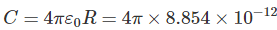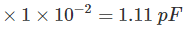Test: Electromagnetic Fields Theory- 2 - Question 4

Which of the following is the unit of electric dipole moment

Detailed Solution for Test: Electromagnetic Fields Theory- 2 - Question 4

The electric dipole moment is a measure of the separation of positive and negative electrical charges. It consists of two equal and opposite charges that are infinitely close together. Its magnitude is equal to the product of the charge and the distance between the charges and having direction from the negative to the positive charge along the line between the charges.

p = qd

The SI unit for electric dipole moment is coulomb-meter

Test: Electromagnetic Fields Theory- 2 - Question 5

Which of the following is generally used to make permanent magnets?

Detailed Solution for Test: Electromagnetic Fields Theory- 2 - Question 5

Materials that can be magnetized, which are also the ones that are strongly attracted to a magnet (ferromagnetic) are used to make a permanent magnet.

Cobalt steel contains about 35% of cobalt. It has a very good magnetic property that is why it is used for making permanent magnets.

Test: Electromagnetic Fields Theory- 2 - Question 6

Copper, Silver, Diamond are examples of:

Detailed Solution for Test: Electromagnetic Fields Theory- 2 - Question 6

Copper, Silver, Diamond are examples of diamagnetic materials.

Diamagnetic materials are slightly repelled by a magnetic field and the material does not retain the magnetic properties when the external field is removed.

Test: Electromagnetic Fields Theory- 2 - Question 7

The dielectric strength of rubber is 30000 V/mm at a frequency of 50 Hz. What is the thickness of insulation required on an electrical conductor at 33 kV to sustain the breakdown?

Detailed Solution for Test: Electromagnetic Fields Theory- 2 - Question 7

Given that,

Electric field (E) = 30000 V/mm

Breakdown voltage (V) = 33 kV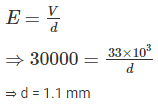Test: Electromagnetic Fields Theory- 2 - Question 8

The λ is the flux linkage of a coil and I is the current through the coil then inductance of the coil is:

Detailed Solution for Test: Electromagnetic Fields Theory- 2 - Question 8

The inductance of a coil is L = λ/I

Where, λ is flux linkage in weber-turns

I is current in amperes

Test: Electromagnetic Fields Theory- 2 - Question 9

The current ratio (i2/i) for the circuit shown in fig. is:Detailed Solution for Test: Electromagnetic Fields Theory- 2 - Question 9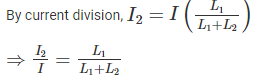Test: Electromagnetic Fields Theory- 2 - Question 10

The direction of the magnetic lines of force around a current carrying conductor can be determined by:

Detailed Solution for Test: Electromagnetic Fields Theory- 2 - Question 10

According to Right-hand thumb rule, imagine that you are holding a current carrying wire in your right hand so that the thumb points in the direction of the current, then the direction in which the fingers wrap the wire will represent the direction of magnetic lines of force.

Test: Electromagnetic Fields Theory- 2 - Question 11

Permeability of the substance is the measure of:

Detailed Solution for Test: Electromagnetic Fields Theory- 2 - Question 11

Permeability is the measure of the ability of a material to support the formation of a magnetic field within itself. It is the degree of magnetization that a material obtains in response to an applied magnetic field.

It is a constant of proportionality that exists between magnetic flux density and magnetic field intensity.

B = μH

The units of magnetic permeability are henry per meter

Test: Electromagnetic Fields Theory- 2 - Question 12

The induced emf in a coil of 0.8 mH carrying 2A current and reversed in 0.4 second is:

Detailed Solution for Test: Electromagnetic Fields Theory- 2 - Question 12

Given that,

Inductance of a coil (L) = 0.8 mH

Current (I) = 2 A

Change in current = 2 – (–2) = 4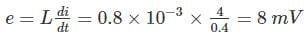Test: Electromagnetic Fields Theory- 2 - Question 13

The differential coupling of two coils in series connection has self-inductance of 2 mH, 4 mH and a mutual inductance of 0.15 mH. The equivalent of the combination is:

Detailed Solution for Test: Electromagnetic Fields Theory- 2 - Question 13

Given that, L1 = 2 mH, L2 = 4 mH, M = 0.15 mH

The equivalent of two coils in series connection for differential coupling is

Leq = L1 + L2 - 2M = 2 + 4 – 2 (0.15) = 5.7 mH

Test: Electromagnetic Fields Theory- 2 - Question 14

The retentivity of a material useful for the construction of:

Detailed Solution for Test: Electromagnetic Fields Theory- 2 - Question 14

This power of magnetism after the applied magnetism is removed is called retentivity of a substance.

Materials which are required to retain their magnetism will have high retentivity and as such are used to make permanent magnets.

Test: Electromagnetic Fields Theory- 2 - Question 15

Higher the self-inductance of a coil

Detailed Solution for Test: Electromagnetic Fields Theory- 2 - Question 15

Self-inductance of a coil is defined as the ratio of self-induced emf to the rate of change of current in the coil.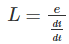Higher the self-inductance of a coil, longer the delay in establishing a steady current through it.

Test: Electromagnetic Fields Theory- 2 - Question 16

The effect of an air gap in a magnetic circuit is to:

Detailed Solution for Test: Electromagnetic Fields Theory- 2 - Question 16

The air gap in magnetic circuit means the magnetic resistance, i.e. reluctance to the magnetic flux density. Hence the air gap indicates the increase in reluctance.

Test: Electromagnetic Fields Theory- 2 - Question 17

What is the MKS rationalized unit of magnetic pole strength quantity?

Detailed Solution for Test: Electromagnetic Fields Theory- 2 - Question 17

Magnetic pole strength is a measure of the force exerted by one face of a magnet on a face of another magnet when both magnets are represented by equal and opposite poles.

Weber is the MKS rationalized unit of magnetic pole strength quantity.

Test: Electromagnetic Fields Theory- 2 - Question 18

When the current in a coil is increased from 2 A to 4 A in 0.05 seconds, the e.m.f. induced in the coil is 8 V. The self-inductance of the coil is

Detailed Solution for Test: Electromagnetic Fields Theory- 2 - Question 18

Given that,

Current (I) = Changed form 2 A to 4 A

Change in current = 2 A

Time (t) = 0.05

Emf (e) = 8 V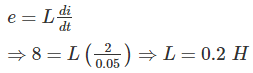Test: Electromagnetic Fields Theory- 2 - Question 19

In the given circuit, inductance L1 and L2, if L1 = 2L2 and Leq is 0.7 H, are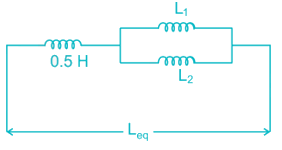Detailed Solution for Test: Electromagnetic Fields Theory- 2 - Question 19

From the given circuit,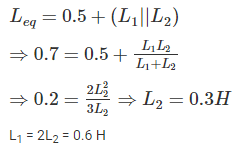Test: Electromagnetic Fields Theory- 2 - Question 20

Soft magnetic materials have

Detailed Solution for Test: Electromagnetic Fields Theory- 2 - Question 20

Soft magnetic materials are those materials that are easily magnetized and demagnetized.

Permeability is the measure of the ability of a material to support the formation of a magnetic field within itself. It is the degree of magnetization that a material obtains in response to an applied magnetic field. Hence it should be high for soft magnetic materials.

Coercive field or coercive force is a measure of the ability of a ferromagnetic material to withstand an external magnetic field without becoming demagnetized. Hence it should be low for soft magnetic materials.

## Mock Test Series for SSC JE Electrical Engineering

2 videos|1 docs|55 tests
Information about Test: Electromagnetic Fields Theory- 2 Page
In this test you can find the Exam questions for Test: Electromagnetic Fields Theory- 2 solved & explained in the simplest way possible. Besides giving Questions and answers for Test: Electromagnetic Fields Theory- 2, EduRev gives you an ample number of Online tests for practice

## Mock Test Series for SSC JE Electrical Engineering

2 videos|1 docs|55 tests